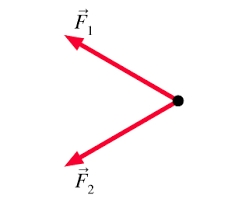# Two forces 3

Two forces of magnitude 8 Newtons and 15 Newtons respectively act at a point. If the resultant force is 17 Newtons, find the angle between the forces.

°Did you find an error or inaccuracy? Feel free to write us. Thank you!Tips to related online calculators
Cosine rule uses trigonometric SAS triangle calculator.